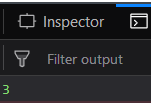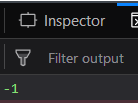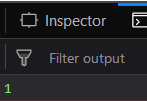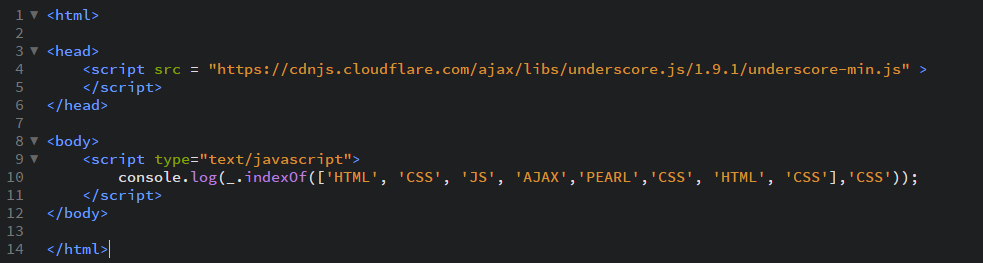Skip to content
Related Articles
Underscore.js | _.indexOf()
• Last Updated : 22 Oct, 2020

_.indexOf() function:

• It gives the index of the elements whose position we need to find.
• It starts to count the position of the elements in the array starting from 0.
• If the element is not present in the array then the result will be -1.

Syntax:

`_.indexOf(array, value, [isSorted])`

Parameters:
It takes three arguments:

• The array
• The value
• The isSorted (optional)

Return value:
It returns the position of the passed element.

Examples:

1. Passing a list of numbers to _.indexOf() function:
The ._indexOf() function takes the element from the list one by one and checks whether it is equal to the passed element in the second parameter. If it is equal then the result is it’s index otherwise -1 is returned.
 `<``html``>``  ` `<``head``>``    ``<``script` `src` `= ``"https://cdnjs.cloudflare.com/ajax/libs/underscore.js/1.9.1/underscore-min.js"` `>``    ``````  ` `<``body``>``    ``<``script` `type``=``"text/javascript"``>``        ``console.log(_.indexOf([1, 2, 3, 4, 5, 6], 4));``    ``````  ` ``

Output:2. Passing a list of characters to the _.indexOf() function:
We can also pass the list of characters to the _.indexOf() function and it will work in the same way as the numbers list works. In the second parameter we need to mention the word whose index we need to find inside single quotes, ”.
 `<``html``>``  ` `<``head``>``    ``<``script` `src` `= ``"https://cdnjs.cloudflare.com/ajax/libs/underscore.js/1.9.1/underscore-min.js"` `>``    ``````  ` `<``body``>``    ``<``script` `type``=``"text/javascript"``>``        ``console.log(_.indexOf(['HTML', 'CSS', ``                  ``'JS', 'AJAX', 'PEARL'], 'AJAX'));``    ``````  ` ``

Output:3. Passing second parameter which is not present in the list::
Pass the list of character elements to the _.indexOf() function. SIince in the given list the second parameter ‘GEEKS’ is not present so the result will be -1.
 `<``html``>``  ` `<``head``>``    ``<``script` `src` `= ``"https://cdnjs.cloudflare.com/ajax/libs/underscore.js/1.9.1/underscore-min.js"` `>``    ``````  ` `<``body``>``    ``<``script` `type``=``"text/javascript"``>``        ``console.log(_.indexOf(['HTML', 'CSS', 'JS',``                  ``'AJAX', 'PEARL'], 'GEEKS'));``    ``````  ` ``

Output:4. Passing a list with repeated elements to the _.indexOf() function: Even tough we pass an array with the repeated element the _.indexOf() function will work in the same way and return the index where the element passed in the second parameter is found first.
 `<``html``>``  ` `<``head``>``    ``<``script` `src` `= ``"https://cdnjs.cloudflare.com/ajax/libs/underscore.js/1.9.1/underscore-min.js"` `>``    ``````  ` `<``body``>``    ``<``script` `type``=``"text/javascript"``>``        ``console.log(_.indexOf(['HTML', 'CSS', 'JS', 'AJAX',``                     ``'PEARL', 'CSS', 'HTML', 'CSS'], 'CSS'));``    ``````  ` ``

Output:NOTE:
These commands will not work in Google console or in firefox as these additional files need to be added which they didn’t have added.
So, add the given links to your HTML file and then run them.
The links are as follows:

 `<``script` `type``=``"text/javascript"` `src` `=``"https://cdnjs.cloudflare.com/ajax/libs/underscore.js/1.9.1/underscore-min.js"``>```

An example is shown below:My Personal Notes arrow_drop_up